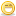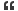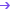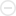1. ## electrical

in a single phase induction motor if the number of poles is 4 frequency is 50hz then what is the speed (RPM) of the motor?Reply With Quote

2. ## Re: electrical

speed= 120f/p
=120X50/4=1500 rpmReply With Quote

3. ## Re: electrical

n=120f/p simple.......Reply With Quote

4. ## Re: electrical

practically i feed 50 hz frequency, for 4 pole induction machine, but i not get 1500 instead i get 1440 RPM, can you please tell, why the droping of rpmReply With Quote

5. ##Re: electrical

Becasue of slip. if it runs on 1500 rpm it will become syn. motor . It is the property of induction motor.Reply With Quote

6. ## Re: electrical

well,n=120*f/p gives the speed of the rotating mmf or the synchronous speed.An induction moor will develop zero torque at this speed and hence will always run at a lower speed(s*n)where s is called the slip.(s is usually in the range .02 to .08 and depends on the load)Reply With Quote

7. ##Re: electrical

f=PNs/120
NS=120f/P
=120*50/4
=1500 rpm

Nr= Rotor Speed will depend on slip.

Nr= Ns(1-s)Reply With Quote

8. ## Re: electrical

how can i select the cable for amps ratingReply With Quote

9. ## Re: electrical

that u can calculate simply by the formulas:

Ns=120*f/p where Ns=speed of the stator ,f =frequency of supply,
and p=no of poles

slip(S)=(Ns-Nr)/Ns

nd rotor frequency fr=S*supply frequency

with the help o fthese formulas you can get wat u want....Reply With Quote

10. ## Re: electrical

cable size primarily depends on its voltage rating or kW
rating of load. From the kW rating, we can calculate the
current that should be carried by cable and the number of
runs required. we also have to calculate voltage drop
during starting and running in case of a motor load.Reply With Quote

11. ## Re: electricalOriginally Posted by zabeerpractically i feed 50 hz frequency, for 4 pole induction machine, but i not get 1500 instead i get 1440 RPM, can you please tell, why the droping of rpm
all things in practice is not ideal,though F=50 Hz. I.M doesnot rotate at 1500RPM by120F/P formula b'use if speed is 1500RPM r,rotor speed =1500RPM,Synchronous flux rotate at 1500RPM ,and EMF induced in the Rotor is zero due to SAME speed hence no induced emf.,no force and Motor will not run.hence slip=Syn.speed-Actual speed =Somthing value Must for rotating motor.Reply With Quote

####Posting Permissions

• You may not post new threads
• You may not post replies
• You may not post attachments
• You may not edit your posts
•